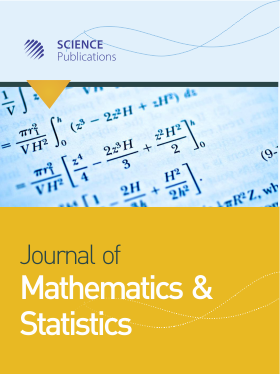Frequency: Continuous
ISSN: 1549-3644 (Print)
ISSN: 1558-6359 (Online)
Research Article Open Access

# Time Series Forecasting by using Seasonal Autoregressive Integrated Moving Average: Subset, Multiplicative or Additive Model

Suhartono 1
• 1 ,
Journal of Mathematics and Statistics
Volume 7 No. 1, 2011, 20-27

DOI:

Submitted On: 29 October 2010 Published On: 29 January 2011

How to Cite: Suhartono, (2011). Time Series Forecasting by using Seasonal Autoregressive Integrated Moving Average: Subset, Multiplicative or Additive Model. Journal of Mathematics and Statistics, 7(1), 20-27. https://doi.org/10.3844/jmssp.2011.20.27

## Abstract

Problem statement: Most of Seasonal Autoregressive Integrated Moving Average (SARIMA) models that used for forecasting seasonal time series are multiplicative SARIMA models. These models assume that there is a significant parameter as a result of multiplication between nonseasonal and seasonal parameters without testing by certain statistical test. Moreover, most popular statistical software such as MINITAB and SPSS only has facility to fit a multiplicative model. The aim of this research is to propose a new procedure for indentifying the most appropriate order of SARIMA model whether it involves subset, multiplicative or additive order. In particular, the study examined whether a multiplicative parameter existed in the SARIMA model. Approach: Theoretical derivation about Autocorrelation (ACF) and Partial Autocorrelation (PACF) functions from subset, multiplicative and additive SARIMA model was firstly discussed and then R program was used to create the graphics of these theoretical ACF and PACF. Then, two monthly datasets were used as case studies, i.e. the international airline passenger data and series about the number of tourist arrivals to Bali, Indonesia. The model identification step to determine the order of ARIMA model was done by using MINITAB program and the model estimation step used SAS program to test whether the model consisted of subset, multiplicative or additive order. Results: The theoretical ACF and PACF showed that subset, multiplicative and additive SARIMA models have different patterns, especially at the lag as a result of multiplication between non-seasonal and seasonal lags. Modeling of the airline data yielded a subset SARIMA model as the best model, whereas an additive SARIMA model is the best model for forecasting the number of tourist arrivals to Bali. Conclusion: Both of case studies showed that a multiplicative SARIMA model was not the best model for forecasting these data. The comparison evaluation showed that subset and additive SARIMA models gave more accurate forecasted values at out-sample datasets than multiplicative SARIMA model for airline and tourist arrivals datasets respectively. This study is valuable contribution to the Box-Jenkins procedure particularly at the model identification and estimation steps in SARIMA model. Further work involving multiple seasonal ARIMA models, such as short term load data forecasting in certain countries, may provide further insights regarding the subset, multiplicative or additive orders.

• 4,359 Views
• 18 Citations

### Keywords

• SARIMA
• multiplicative parameter